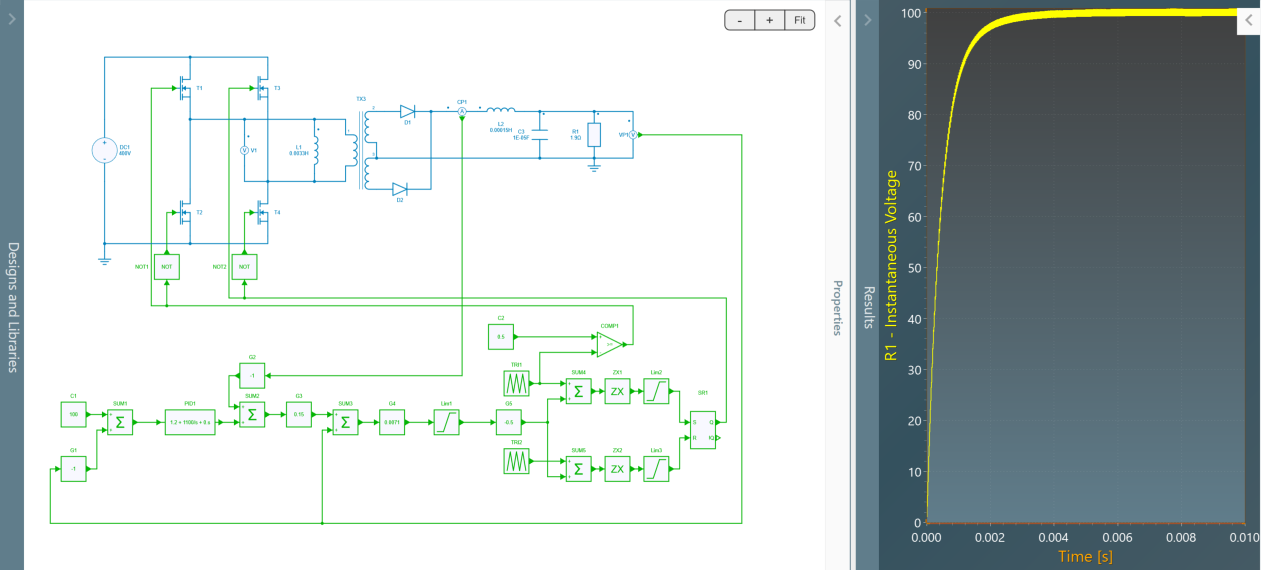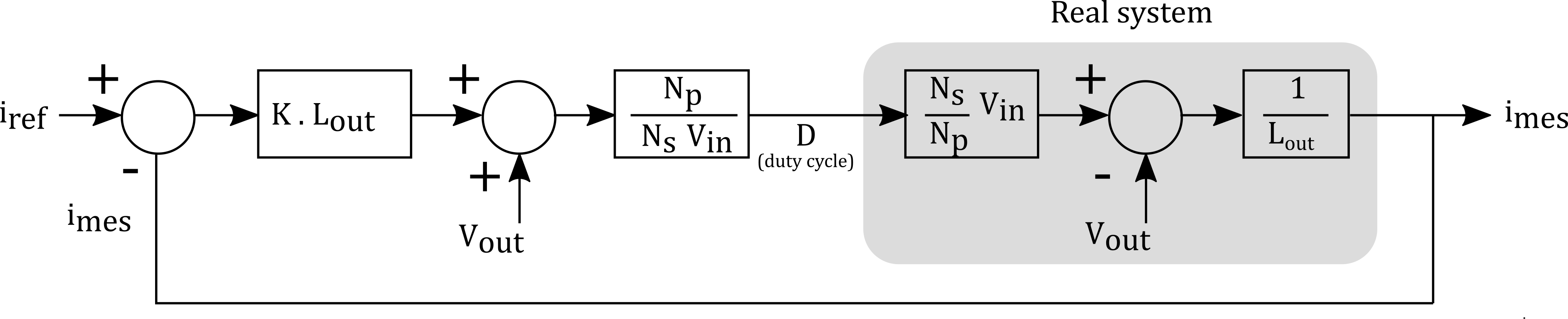Resources

# DC/DC Phase Shift Full Bridge

This example shows a Phase-Shift Full Bridge converter with:

• an input voltage of 400 V,
• an output voltage of 100 V,
• a power of 5.3 kW.

In this example, the transformer is supposed to have one primary winding and two secondary windings. As multi-windings are not available in Simba yet, two transformers are used: the primary windings are connected in parallel to behave like a single winding and the secondary windings are connected in series.## Phase-shift PWM

Each switching cell is driven with a duty cycle of 50%. The control signal of the phase-shifted switching cell is created from the duty cycle reference according to the figure below. The principle is to compare the duty cycle reference with a sawtooth to determine the phase-shift between the two legs.Each switching cell shows its own duty cycle set at 50 %.

1. at $t=0$, the first switching cell is turned on;
2. the duty cycle value is first divided by 2;
3. this value is compared to a first sawtooh (black) between 0 and 1 at the switching frequency;
4. when the sawtooth reaches this value, the second switching cell is turned on;
5. at $t=T_{sw}/2$, the first swithing cell is turned off;
6. when the second sawtooth (orange) - delayed of $Tsw/2$ with the first one - reaches the value of the duty cycle half, the second switching cell is turned off.

Then the differential voltage between the two switching cells shows 4 phases during a switching period:

1. E during a time lapse equal to $D T_{sw} / 2$;
2. 0 during time lapse equal to $(1-D) T_{sw} / 2$;
3. -E during a time lapse equal to $D T_{sw} / 2$;
4. 0 during time lapse equal to ($(1-D) T_{sw} / 2$.

## Current loop

This current loop implements an output voltage compensation to simplify the open-loop transfer function and to only keep the integrator behavior of the inductance. Then a simple gain correction can be used.## Voltage loop

The voltage loop involves a classic PI regulator.